# Rotational Kinematics

## IntroductionThe goal: to re-express our kinematics, dynamics, and forces in terms of rotating objects.

We'll have to use angular displacements instead of linear ones.

## Rotational Motion VariablesLinear Velocity, $v$, referred to motion in $x$ or $y$ directions (or a combination).

Angular velocity, $\omega$, refers to the motion of an object around a center point.

$$\overline{\omega} = \frac{\Delta \theta}{\Delta t}$$
$$\overline{\omega} = \frac{\theta_f-\theta_i}{t_f-t_i}$$
$$\omega = \frac{d\theta}{dt}$$

This will be easier in the long run, although it takes some getting used to.

What is the angular velocity of the record player?

#### Angular Acceleration

Of course, $\omega$ can change.

If that happens then we'll have to use an angular acceleration to describe that motion.

$$\overline{\alpha} = \frac{\Delta \omega}{\Delta t}$$
$$\alpha = \frac{d\omega}{dt}$$

#### Sign Conventions

Choosing a positive and negative was easy in linear motion.

For angular velocities, we need a convention:From this, we can also declare the sign of accleration:## Angular Velocity Vector

To describe the direction of an angular velocity, we'll need the Right Hand Rule.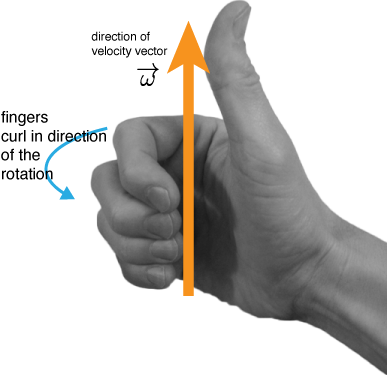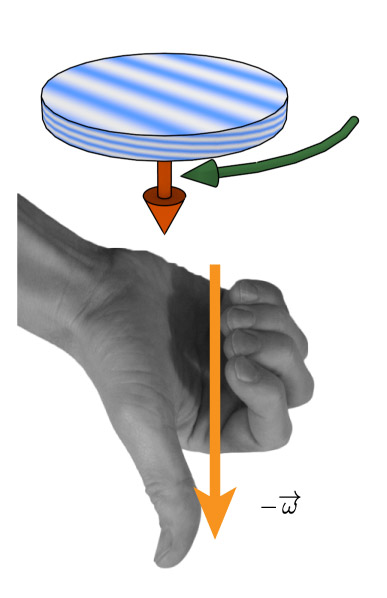In the case of a negative $\omega$, the same right hand rule can help you out.

This wheel is rotating at constant angular velocity $\omega$ in the direction of the blue arrow. What vector describes the direction of the angular velocity vector?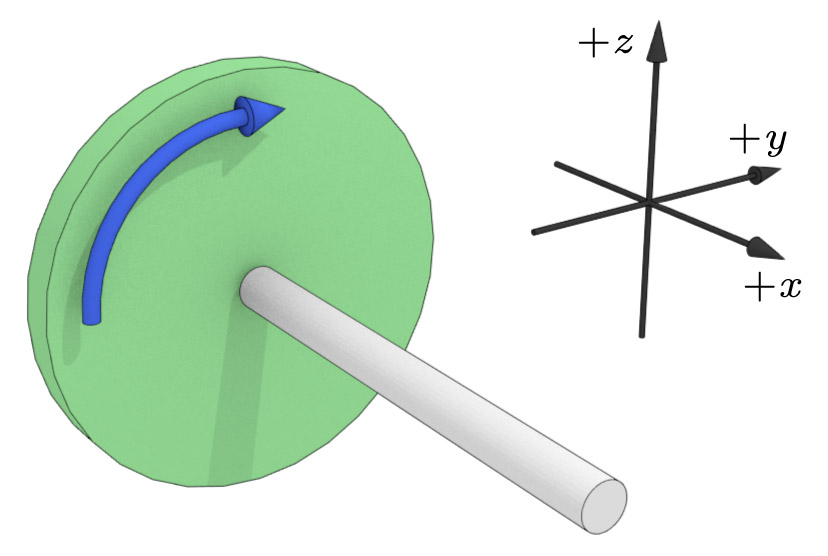1. $+\mathbf{\hat{i}}$
2. $-\mathbf{\hat{i}}$
3. $+\mathbf{\hat{j}}$
4. $-\mathbf{\hat{j}}$
5. $+\mathbf{\hat{k}}$
6. $-\mathbf{\hat{k}}$
1. $+\mathbf{x}$
2. $-\mathbf{x}$
3. $+\mathbf{y}$
4. $-\mathbf{y}$
5. $+\mathbf{z}$
6. $-\mathbf{z}$

The wheel is rotating in the direction of the blue arrow, but is slowing down. Which direction is the angular acceleration vector pointing?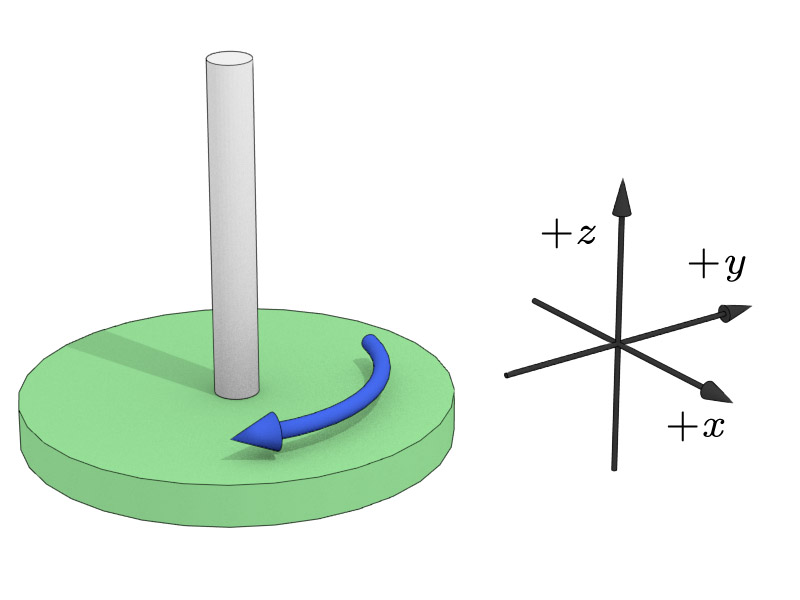1. $+\mathbf{\hat{i}}$
2. $-\mathbf{\hat{i}}$
3. $+\mathbf{\hat{j}}$
4. $-\mathbf{\hat{j}}$
5. $+\mathbf{\hat{k}}$
6. $-\mathbf{\hat{k}}$
1. $+\mathbf{x}$
2. $-\mathbf{x}$
3. $+\mathbf{y}$
4. $-\mathbf{y}$
5. $+\mathbf{z}$
6. $-\mathbf{z}$

The wheel is rotating in the direction of the blue arrow, but is speeding up. Which direction is the angular acceleration vector pointing?1. $+\mathbf{\hat{i}}$
2. $-\mathbf{\hat{i}}$
3. $+\mathbf{\hat{j}}$
4. $-\mathbf{\hat{j}}$
5. $+\mathbf{\hat{k}}$
6. $-\mathbf{\hat{k}}$
1. $+\mathbf{x}$
2. $-\mathbf{x}$
3. $+\mathbf{y}$
4. $-\mathbf{y}$
5. $+\mathbf{z}$
6. $-\mathbf{z}$

A wheel rotates at some angular velocity...

## Rotational Kinematics

Fortunately, they are all the same!

Equations for linear motion Equations for angular motion
$$v = v_{0} + a t$$ $$\omega = \omega_{0} + \alpha t$$
$$x = \frac{(v + v_{0})t}{2}$$ $$\theta = \frac{(\omega + \omega_{0})t}{2}$$
$$x =x_0 + v_{0} t + \frac{a t^2}{2}$$ $$\theta =\theta_0+ \omega_{0} t + \frac{\alpha t^2}{2}$$
$$v^2 = v_{0}^2 + 2a_x x$$ $$\omega^2 = \omega_{0}^2 + 2\alpha \theta$$

An old fashioned computer disk spins up to 5400 rpm in 2.0 s. Find the angular acceleration of the disc. After two seconds, how many revolutions has it made?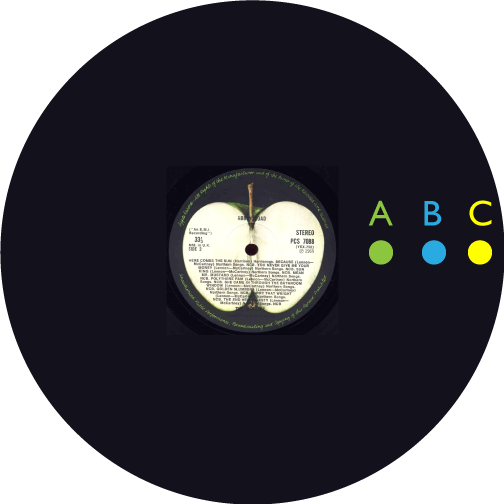Which point on this record has the largest angular velocity ($\omega$)?

1. A
2. B
3. C
4. They are all equal

The propeller of an airplane is at rest when the pilot starts the engine; and its angular acceleration is a constant value. Two seconds later, the propeller is rotating at 10$\pi$ rad/s. Through how many revolutions has the propeller rotated through during the first two seconds?We can ask about the tangential velocity or speed of an object going around in circles.

Since $s = r \theta$ (in radians):

$$\frac{s}{t} = \frac{r \theta}{t} = r\omega$$

which will give us a tangential velocity: $v_T$.

$$v_T = r \omega$$Similarly, we can ask about the tangential angular acceleration in the same way:

$$a_T = \frac{v_T - v_{T0}}{t} = \frac{r \omega - r \omega_0}{t} = r \left(\frac{\omega - \omega_0}{t}\right)$$
$$a_T = r \alpha$$

Which vectors are being displayed here?

1. Angular velocity, $\omega$
2. Tangential velocity, $v_T$
3. Centripetal acceleration, $a_c$
4. Tangential acceleration, $a_T$
5. Angular acceleration, $\alpha$

#### Rolling ObjectsIf an object is rolling, then it will undergo both rotational motion and translational motion.

#### Rolling ObjectWhile the tire is rolling, the arc length $s$ will be traced out along the ground.

If we consider the distance traveled by the tire, $d$, we can see that it must be the same as $s$. Thus:

$$v_{cm} = r \omega$$

likewise for the acceleration:

$$a_{cm} = r \alpha$$

This condition is often called rolling without slipping.

A bicycle is moving forward with a speed $v_0$. At any moment in time, what is speed of the lowermost point of one of the wheels?

#### Rolling without slipping#### Rolling

A point at the rim of a rolling wheel, when not slipping at all, will trace out the following curve:It's known as a cycloid.

#### Angular momentum

Linear momentum was given by the mass times the velocity: $$p = mv$$

Angular momentum will be given by the analogous terms: $$L = I \omega$$

## The Cross Product

The cross product (or vector product) produces another vector:

2. The cross product (or vector product)

$|\mathbf{a} \times \mathbf{b}| = a b \sin \phi$

This produces a third vector that points perpendicular to both the original vectors.

$\mathbf{a} \times \mathbf{b} = (a_x \hat{\mathbf{i}}+a_y \hat{\mathbf{j}}+a_z \hat{\mathbf{k}})\times(b_x \hat{\mathbf{i}}+b_y \hat{\mathbf{j}}+b_z \hat{\mathbf{k}})$These are basic definitions of a right handed coordinate system:

$$\hat{\mathbf{i}} \times \hat{\mathbf{i}} = 0$$ $$\hat{\mathbf{j}} \times \hat{\mathbf{j}} = 0$$ $$\hat{\mathbf{k}} \times \hat{\mathbf{k}} = 0$$
\begin{aligned} \hat{\mathbf{i}} \times \hat{\mathbf{j}} & = \hat{\mathbf{k}}\\ \hat{\mathbf{j}} \times \hat{\mathbf{i}} & = -\hat{\mathbf{k}}\\ \hat{\mathbf{k}} \times \hat{\mathbf{i}} & = \hat{\mathbf{j}}\\ \hat{\mathbf{i}} \times \hat{\mathbf{k}} & = -\hat{\mathbf{j}}\\ \hat{\mathbf{j}} \times \hat{\mathbf{k}} & = \hat{\mathbf{i}}\\ \hat{\mathbf{k}} \times \hat{\mathbf{j}} & = -\hat{\mathbf{i}}\\ \end{aligned}

We can calculate the cross product by computing the determinant of this matrix:

$$\mathbf{A} \times \mathbf{B} = \begin{vmatrix} \mathbf{i} & \mathbf{j} & \mathbf{k} \\ A_x & A_y & A_z \\ B_x & B_y & B_z \\ \end{vmatrix}$$

All said and done, it looks like this:

$$\mathbf{A} \times \mathbf{B} = (A_y B_z-A_z B_y)\hat{\mathbf{i}}+(A_z B_x-A_x B_z)\hat{\mathbf{j}}+(A_x B_y-A_y B_x)\hat{\mathbf{k}}$$

This is another vector!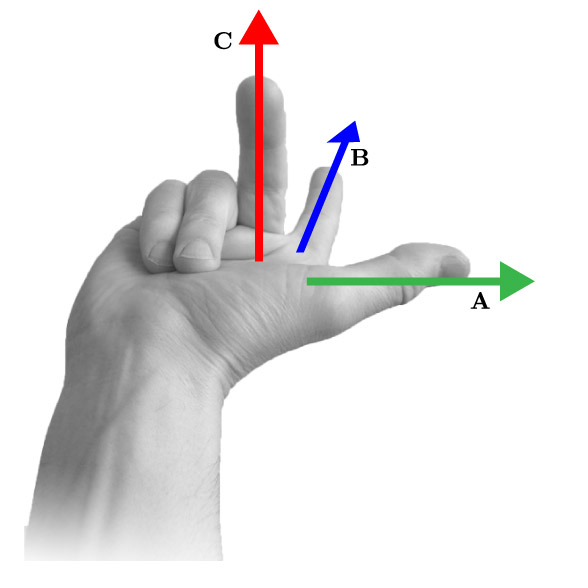The Right-Hand Rule can be used to gain qualitative sense of direction when performing cross products.

$$\mathbf{A} \times \mathbf{B} = \mathbf{C}$$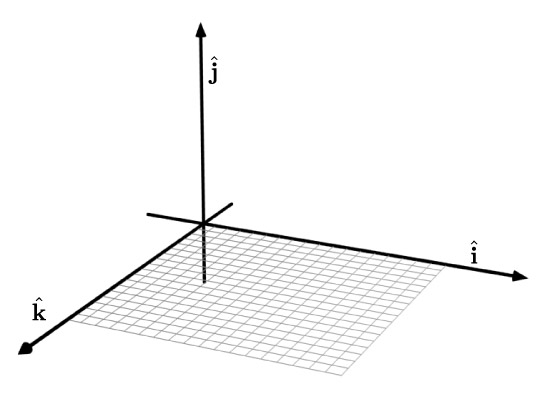$$\hat{\mathbf{i}} \times \hat{\mathbf{j}} = \hat{\mathbf{k}}$$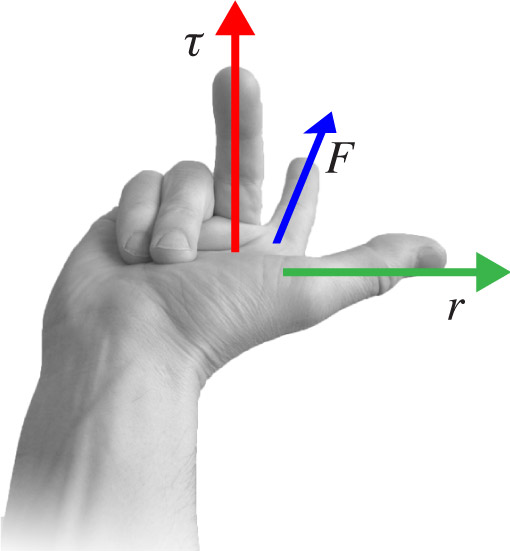The Right-Hand Rule, (version 2) can be used to gain qualitative sense of direction when performing cross products.

Put your thumb in the direction of $r$, then your index finger in the direction of $F$. Torque will point in direction that your upright middle finger points.

The cross product:Which of the following coordinate systems is (are) a valid right hand coordinate system?If $$\mathbf{A}= -2 \mathbf{\hat{i}} + 2 \mathbf{\hat{j}} - 3 \mathbf{\hat{k}}$$ and $$\mathbf{B}= -1 \mathbf{\hat{i}} +1 \mathbf{\hat{j}} + 2 \mathbf{\hat{k}}$$ find $$\mathbf{A}\times \mathbf{B}$$.

Evaluate the following vector product:
If $$\mathbf{A}= -1 \mathbf{\hat{i}} + 3 \mathbf{\hat{j}} - 2 \mathbf{\hat{k}}$$ and $$\mathbf{B}= +3 \mathbf{\hat{i}} -2 \mathbf{\hat{j}} + 4 \mathbf{\hat{k}}$$ find $$\mathbf{A}\times \mathbf{B}$$.